Learn all Concepts of Chapter 3 Class 10 (with VIDEOS). Check - Linear Equations in 2 Variables - Class 10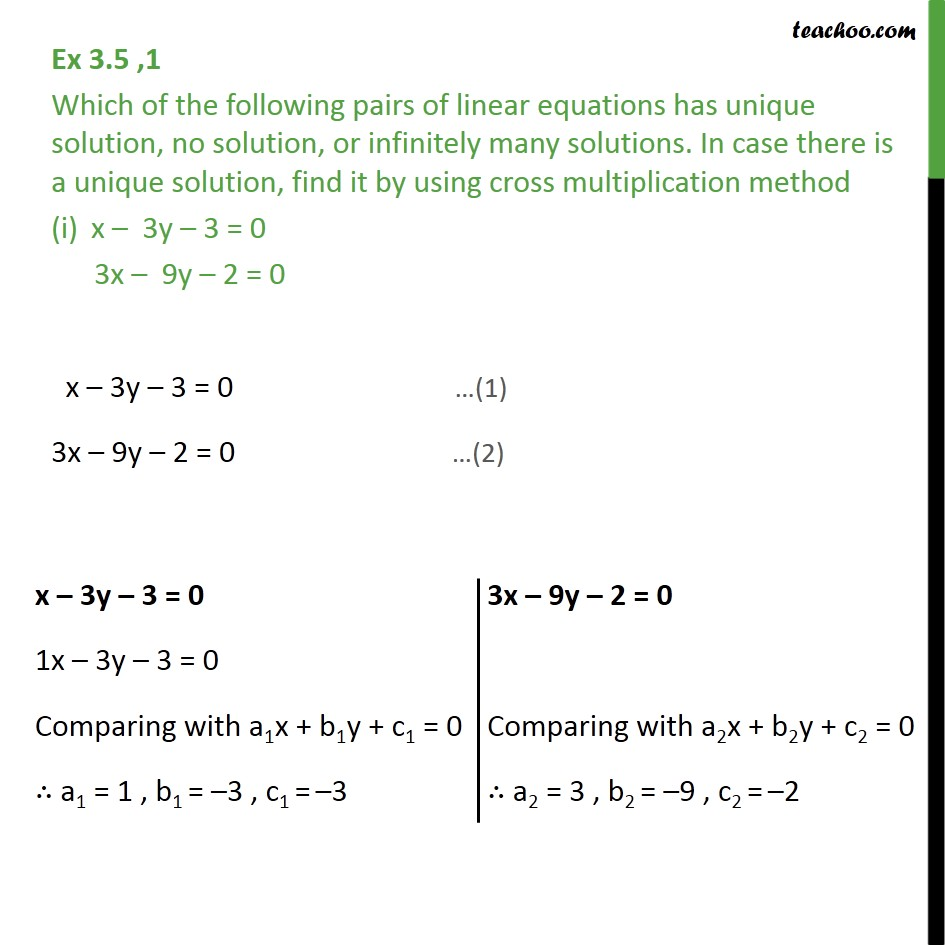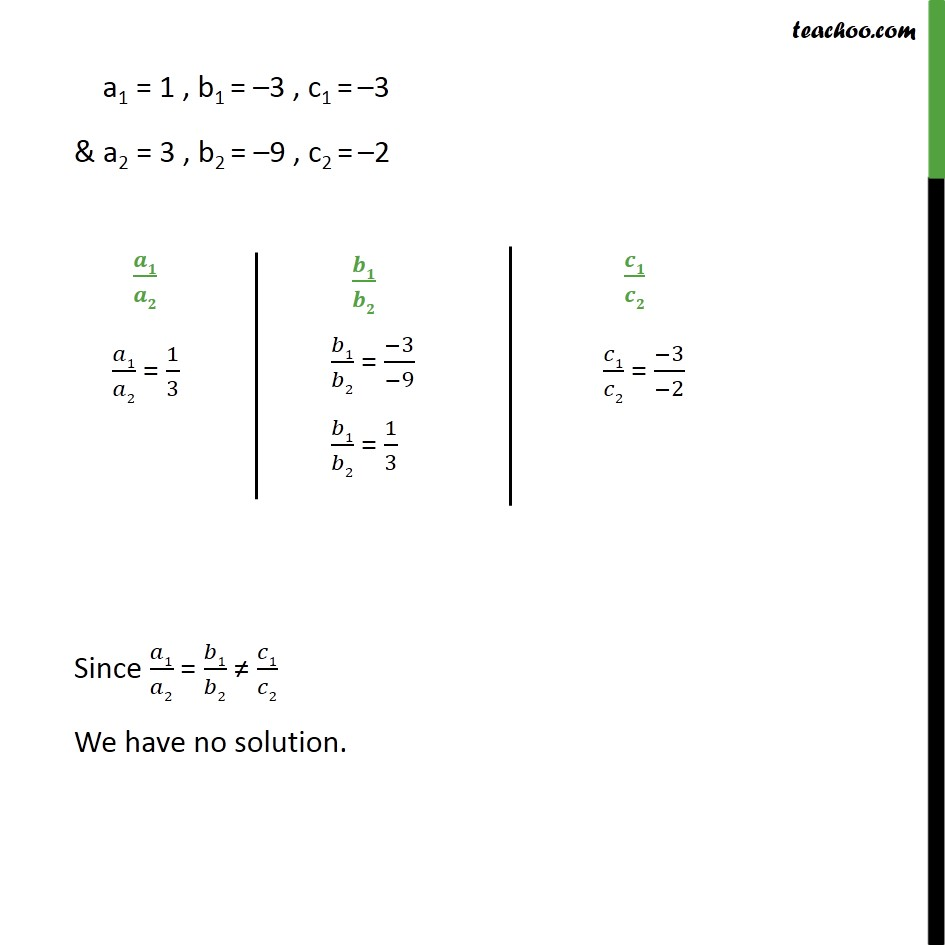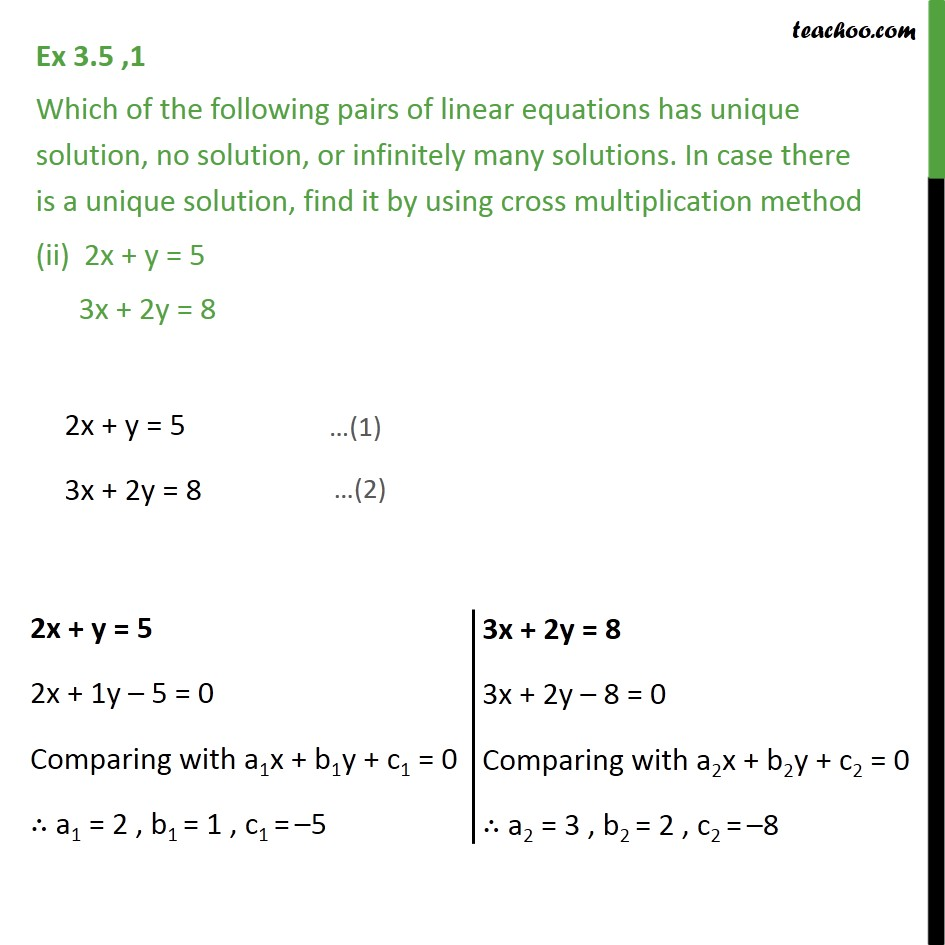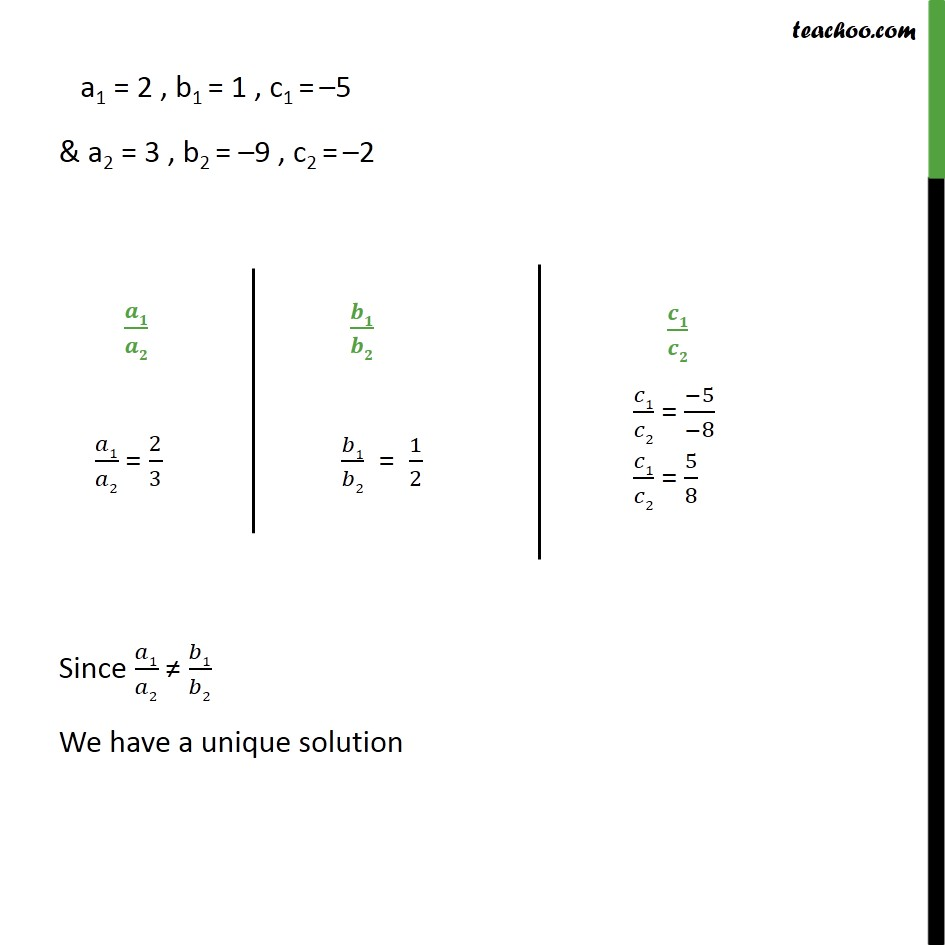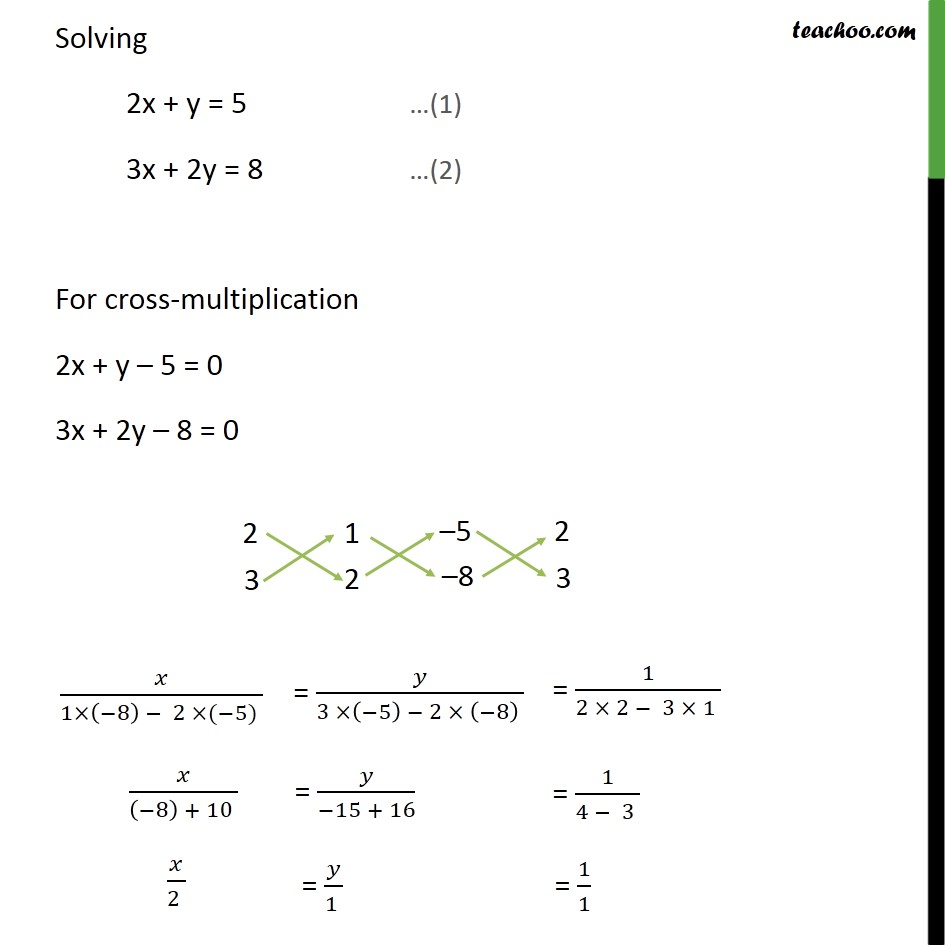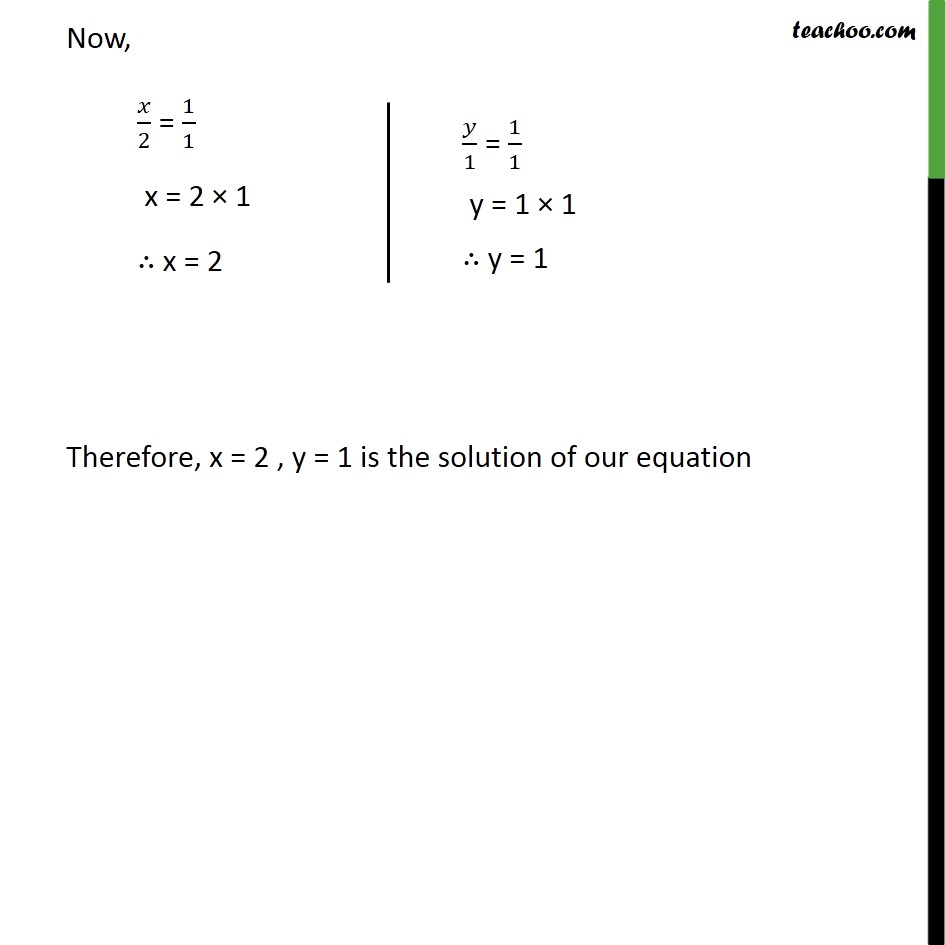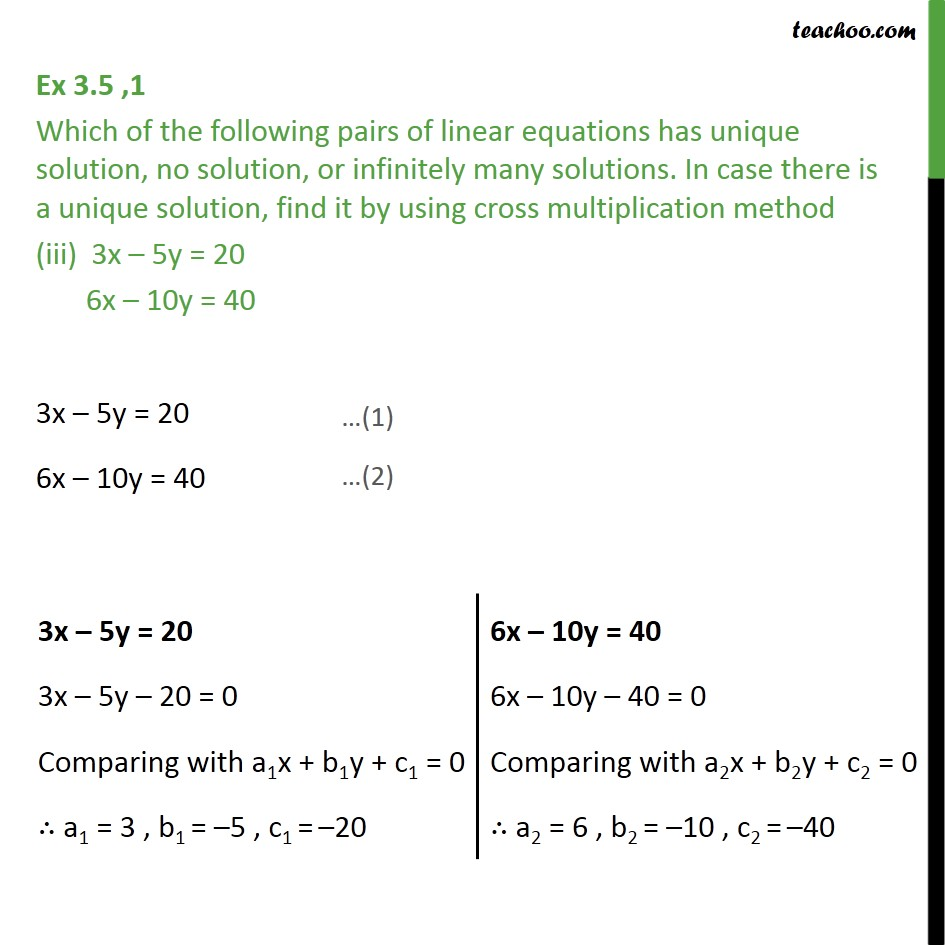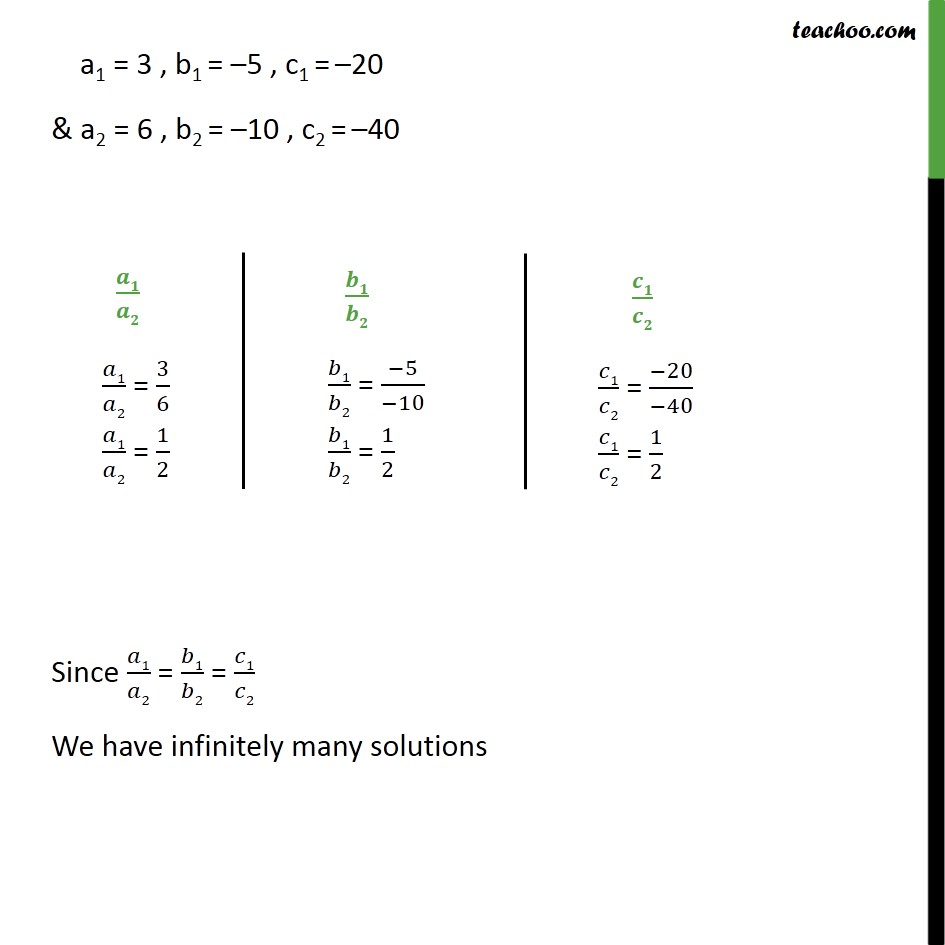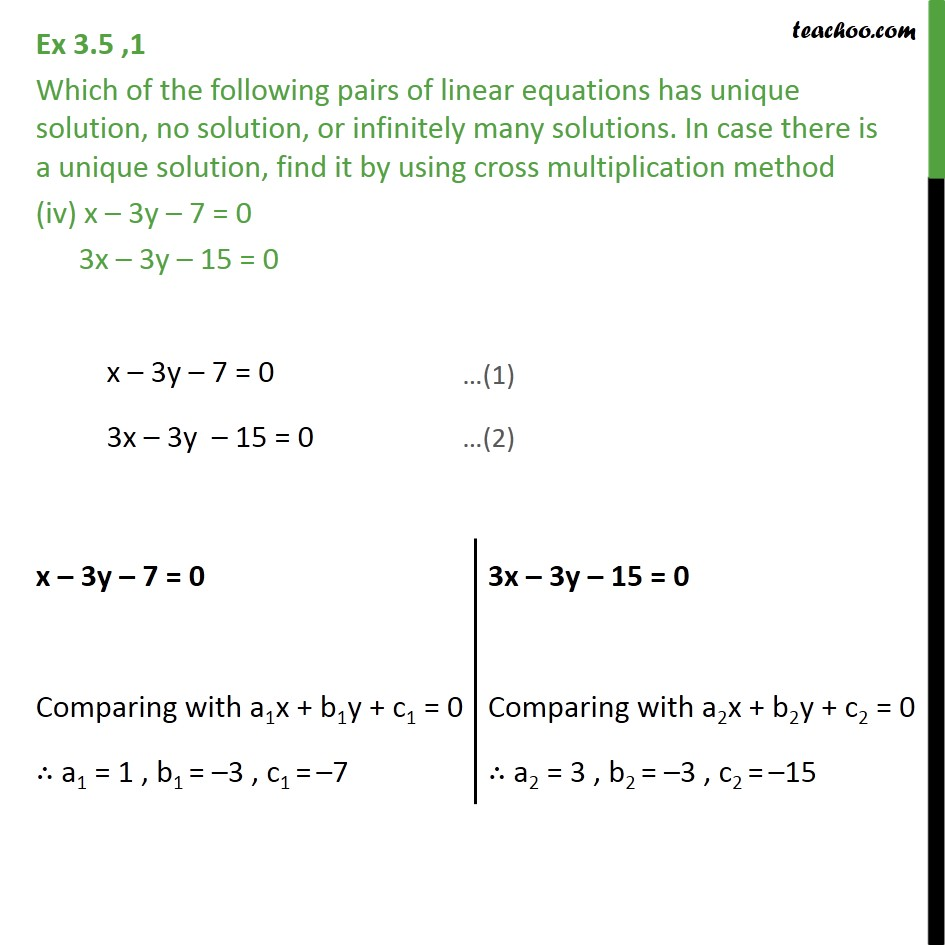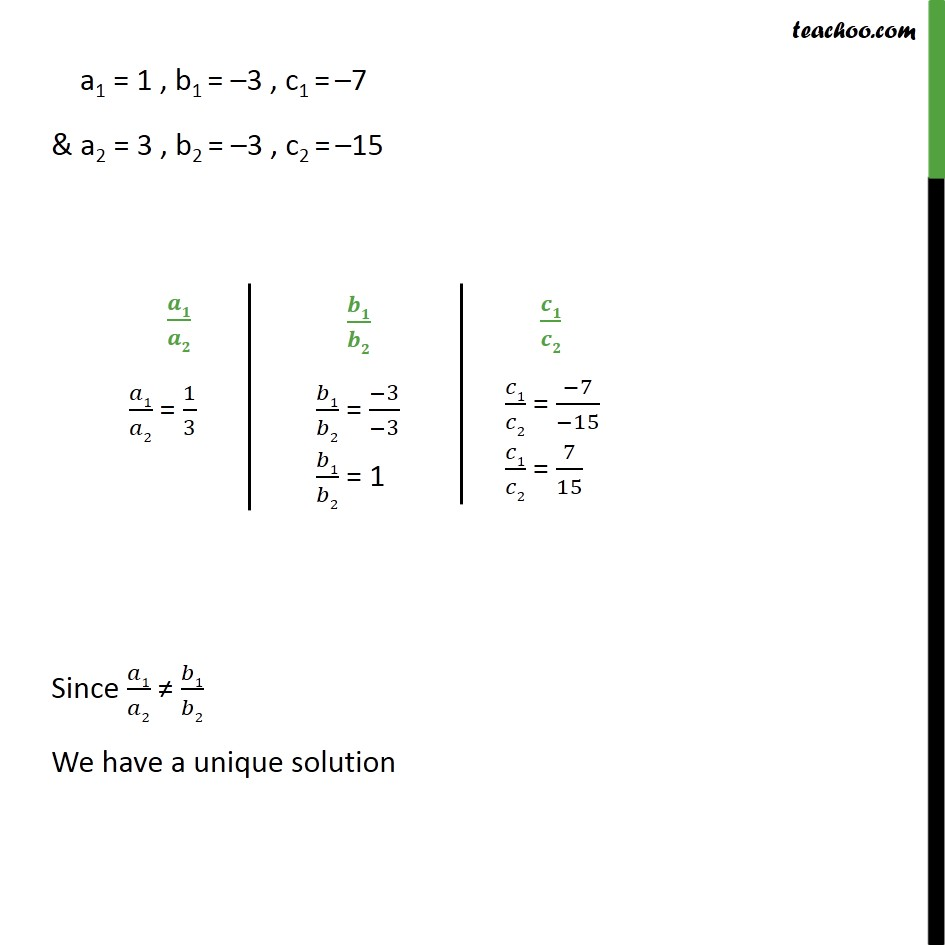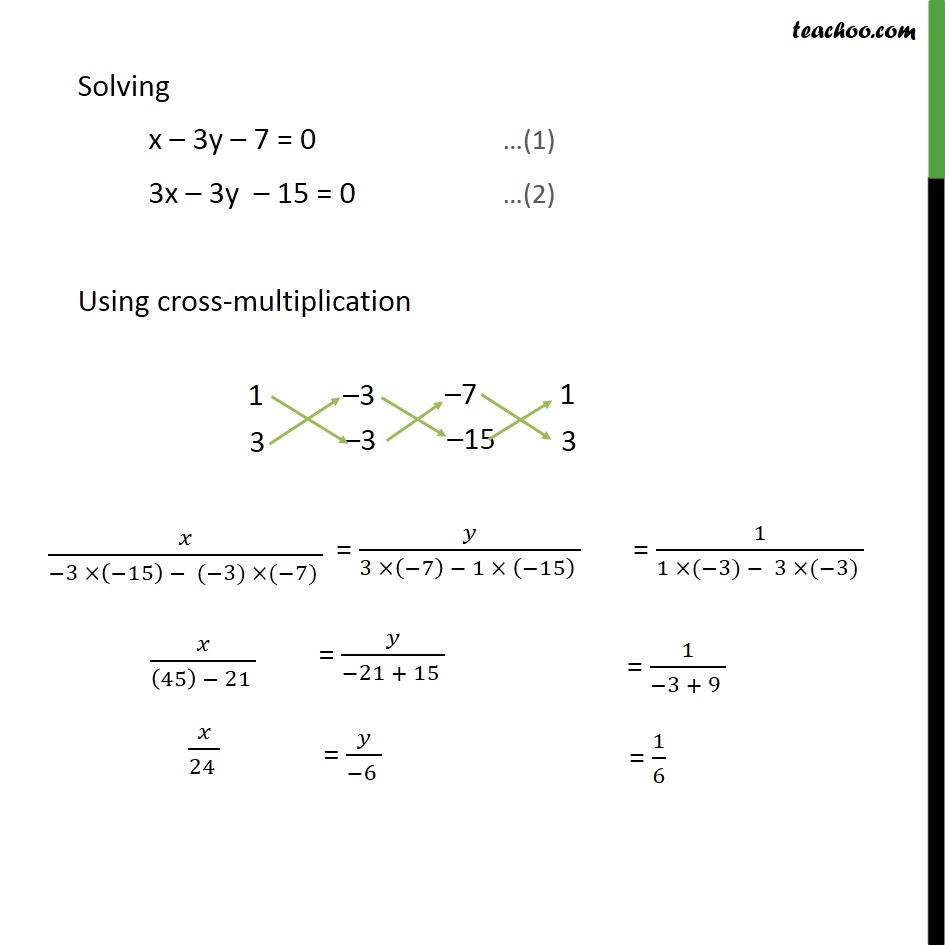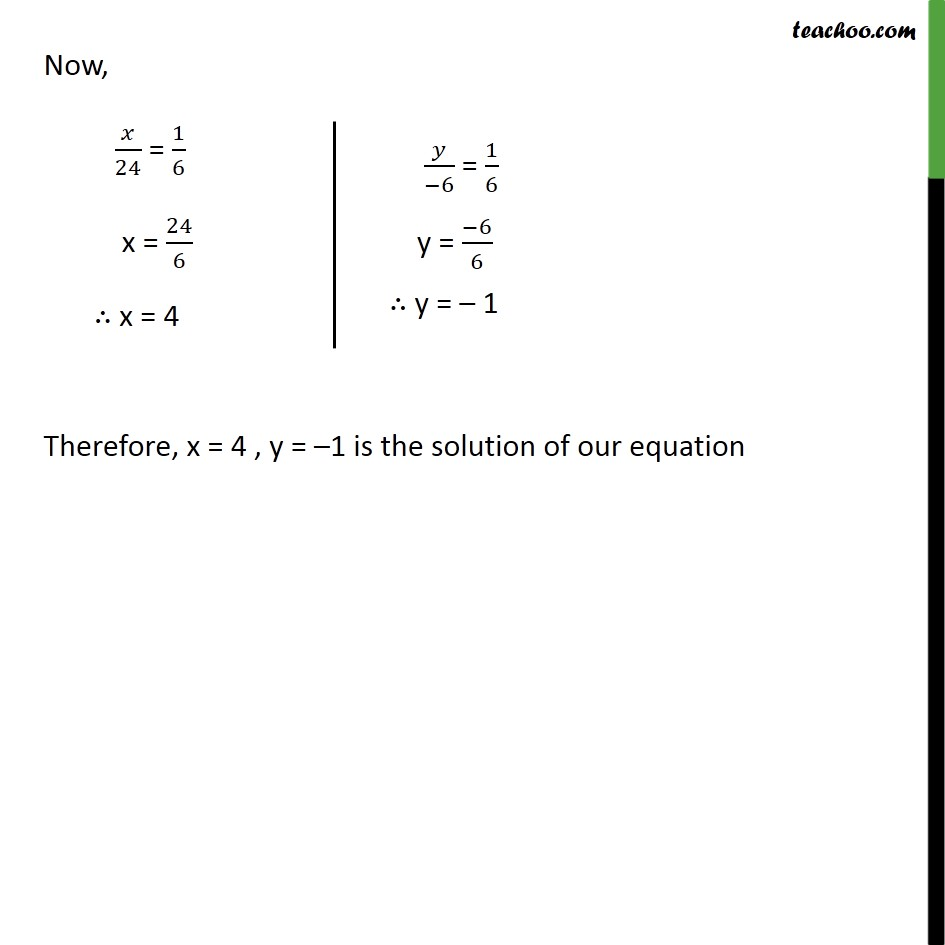1. Chapter 3 Class 10 Pair of Linear Equations in Two Variables
2. Serial order wise
3. Ex 3.5

Transcript

Ex 3.5 ,1 Which of the following pairs of linear equations has unique solution, no solution, or infinitely many solutions. In case there is a unique solution, find it by using cross multiplication method x – 3y – 3 = 0 3x – 9y – 2 = 0 x – 3y – 3 = 0 3x – 9y – 2 = 0 a1 = 1 , b1 = –3 , c1 = –3 & a2 = 3 , b2 = –9 , c2 = –2 Since 𝑎1/𝑎2 = 𝑏1/𝑏2 ≠ 𝑐1/𝑐2 We have no solution. Ex 3.5 ,1 Which of the following pairs of linear equations has unique solution, no solution, or infinitely many solutions. In case there is a unique solution, find it by using cross multiplication method (ii) 2x + y = 5 3x + 2y = 8 2x + y = 5 3x + 2y = 8 a1 = 2 , b1 = 1 , c1 = –5 & a2 = 3 , b2 = –9 , c2 = –2 Since 𝑎1/𝑎2 ≠ 𝑏1/𝑏2 We have a unique solution Solving 2x + y = 5 …(1) 3x + 2y = 8 …(2) For cross-multiplication 2x + y – 5 = 0 3x + 2y – 8 = 0 Now, 𝑥/2 = 1/1 x = 2 × 1 ∴ x = 2 Therefore, x = 2 , y = 1 is the solution of our equation Ex 3.5 ,1 Which of the following pairs of linear equations has unique solution, no solution, or infinitely many solutions. In case there is a unique solution, find it by using cross multiplication method (iii) 3x – 5y = 20 6x – 10y = 40 3x – 5y = 20 6x – 10y = 40 a1 = 3 , b1 = –5 , c1 = –20 & a2 = 6 , b2 = –10 , c2 = –40 Since 𝑎1/𝑎2 = 𝑏1/𝑏2 = 𝑐1/𝑐2 We have infinitely many solutions Ex 3.5 ,1 Which of the following pairs of linear equations has unique solution, no solution, or infinitely many solutions. In case there is a unique solution, find it by using cross multiplication method (iv) x – 3y – 7 = 0 3x – 3y – 15 = 0 x – 3y – 7 = 0 3x – 3y – 15 = 0 a1 = 1 , b1 = –3 , c1 = –7 & a2 = 3 , b2 = –3 , c2 = –15 Since 𝑎1/𝑎2 ≠ 𝑏1/𝑏2 We have a unique solution Solving x – 3y – 7 = 0 …(1) 3x – 3y – 15 = 0 …(2) Using cross-multiplication Now, 𝑥/24 = 1/6 x = 24/6 ∴ x = 4 Therefore, x = 4 , y = –1 is the solution of our equation

Ex 3.5